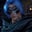Related Tags

communitycreator
javascript

# Round off a number to the next multiple of 5 using JavaScriptGutha Vamsi Krishna

In this shot, we will learn how to round off a number to the next multiple of 5 using JavaScript.

### Problem

Given a positive integer i, round i to the next whole number divisible by 5.

#### Example 1

Input: 47

Output: 50

Explanation: 47 is not divisible by 5, and the next whole number divisible by 5 after 47 is 50.

#### Example 2

Input: 24

Output: 25

Explanation: 24 is not divisible by 5, and the next whole number divisible by 5 after 24 is 25.

### Solution

• Take the given variable i.
• Divide variable i with integer 5.
• Calculate the ceil of the above result.
• Multiple it with 5.
• It will give the next whole number divisible by 5 after variable i.

### Implementation

#### Example 1

//given number
let i = 47;

//calculate next whole number divisible by 5
let nextNum = Math.ceil(i/5) * 5;

//print next number
console.log(nextNum);

#### Explanation

In the above code snippet:

• In line 2: We initialize integer i with value 47.
• In line 5: We divide i with 5 and take the ceil of it. Then, we multiply it with 5 and assign it to the variable nextNum.
• In line 8: We print variable nextNum to the console which is the next whole number divisible by 5 after the given number 47.

### Example 2

//given number
let i = 24;

//calculate next whole number divisible by 5
let nextNum = Math.ceil(i/5) * 5;

//print next number
console.log(nextNum);

#### Explanation

In the above code snippet:

• In line 2: We initialize integer i with value 24.
• In line 5: We divide i with 5 and take the ceil of it. Then, we multiply it with 5 and assign it to the variable nextNum.
• In line 8: We print the variable nextNum to the console, which is the next whole number divisible by 5 after the given number 24.

RELATED TAGS

communitycreator
javascript

CONTRIBUTORGutha Vamsi Krishna
RELATED COURSES

View all Courses

Keep Exploring

Learn in-demand tech skills in half the time